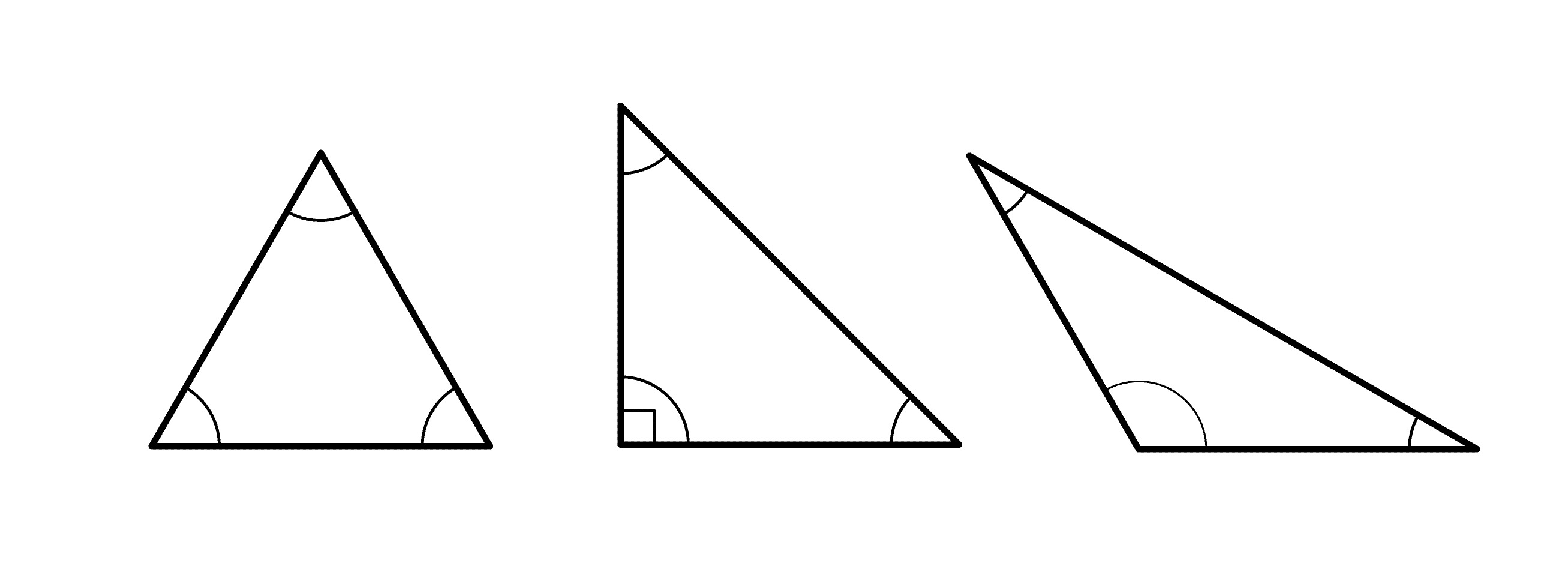# RIGHT TRIANGLE and SPECIAL RIGHT TRIANGLES

With a look through the book, many will recall the Pythagorean Theorem and be able to slowly get their child through the first few problems. But then the special right triangles appear and Geometry gets a bit more complicated… but don’t worry, a great tutor can help explain right triangles to your child and help them succeed in Geometry!# RIGHT TRIANGLE and SPECIAL RIGHT TRIANGLES

Last Updated on September 30, 2020 by A Plus In-Home Tutors

# Many parents in Laguna Hills can explain a right triangle to their children in Geometry.

With a look through the book, many will recall the Pythagorean Theorem and be able to slowly get their child through the first few problems.  But then the special right triangles appear and Geometry gets a bit more complicated… but don’t worry, a great tutor can help explain right triangles to your child and help them succeed in Geometry!

## Geometry Review

A right triangle is a triangle where one of the angles is a right (90 degree) angle.  Think about the intersection of two street corners in Tustin – that’s a right angle.  The top of shopping centers in Tustin?  There are right angles there, too!

### In order to find the sides of a right triangle, a Geometry Tutor will start by explaining the famous Pythagorean Theorem:

A2+ B2 = C2

In Geometry, A and B are the legs of the triangle (AKA, the ones that touch the right angle.)  C is the hypotenuse of the right triangle (the longest side, AKA the diagonal one.)  If you have any two sides of the triangle, you can use this Geometry formula to find the third side.

There are two special right triangles that a Geometry teacher may ask a student to memorize.  It’s much easier to find the sides of these triangles through memorization than using the Pythagorean Theorem.  These are:

### 45–45–90 triangle, which looks like this:

The sides in this triangle are in the ratio 1 : 1 : √2.  You can quickly identify this triangle because it is Isosceles (the two legs are equal) or because the angles are equal (both 45 degrees.) A Geometry tutor in Laguna Hills might explain this as looking for “Side, side, side radical two.”

### 30–60–90 triangle

This is a triangle whose three angles are in the ratio 1 : 2 : 3 and measure 30°, 60°, and 90°. The sides are in the ratio 1 : √3 : 2.  A Geometry tutor in Tustin would explain this as “side, side radical three, twice the side.”